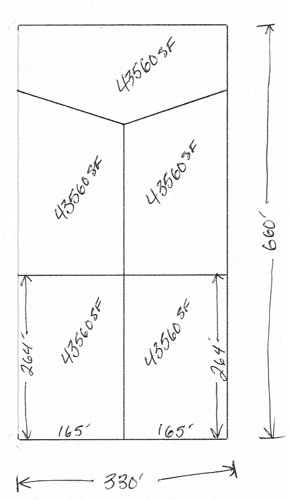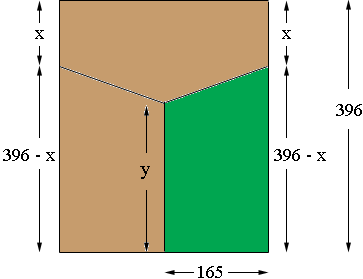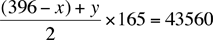SEARCH HOMEMath Central Quandaries & QueriesSubject: finding the perimeter of an irregular pentagon Name: John Who are you: Other I have a 5 acre piece of property that I wish to split into 5 individual 1 acre parcels. The 5 acre parcel 1s 660 feet in length and 330 feet in width. The first two parcels are 264'x165', but I am asking for help getting the perimeters of the last 3 lots, one of which is a irregular pentagon. All of the one acre parcels must have an area of 43,560 square feet each. Can anyone help me? Thanks, JohnHi John,

I reproduced the drawing you sent.You have a choice to make because there is more than one answer. I redrew the top three parcels and labeled some lengths, in feet.I want to concentrate on one of the parcels, the one shaded green. If x and y are such that this parcel has an area of one acre then the lot beside it will also have an area of one acre leaving one acre for the parcel with a pentagon shape. The green parcel has the shape of a trapezoid and the area of a trapezoid is the average of the lengths of the parallel sides times the distance between the parallel sides. Thus if this parcel has an area of one acre (43560 square feet) thenSimplifying this equation gives

y = 132 + x

Thus if you decide on a value for x then y is determined by y = 132 - x and each of the three parcels has an area of 1 acre.

PennyMath Central is supported by the University of Regina and The Pacific Institute for the Mathematical Sciences.